Want to share your content on R-bloggers? click here if you have a blog, or here if you don't.

Seventh post of our series on classification from scratch. The latest one was on the neural nets, and today, we will discuss SVM, support vector machines.

## A formal introduction

Here $y$ takes values in $\{-1,+1\}$. Our model will be $$m(\mathbf{x})=\text{sign}[\mathbf{\omega}^T\mathbf{x}+b]$$ Thus, the space is divided by a (linear) border$$\Delta:\lbrace\mathbf{x}\in\mathbb{R}^p:\mathbf{\omega}^T\mathbf{x}+b=0\rbrace$$

The distance from point $\mathbf{x}_i$ to $\Delta$ is $$d(\mathbf{x}_i,\Delta)=\frac{\mathbf{\omega}^T\mathbf{x}_i+b}{\|\mathbf{\omega}\|}$$If the space is linearly separable, the problem is ill posed (there is an infinite number of solutions). So consider
$$\max_{\mathbf{\omega},b}\left\lbrace\min_{i=1,\cdots,n}\left\lbrace\text{distance}(\mathbf{x}_i,\Delta)\right\rbrace\right\rbrace$$The strategy is to maximize the margin. One can prove that we want to solve $$\max_{\mathbf{\omega},m}\left\lbrace\frac{m}{\|\mathbf{\omega}\|}\right\rbrace$$
subject to $y_i\cdot(\mathbf{\omega}^T\mathbf{x}_i)=m$, $\forall i=1,\cdots,n$. Again, the problem is ill posed (non identifiable), and we can consider $m=1$: $$\max_{\mathbf{\omega}}\left\lbrace\frac{1}{\|\mathbf{\omega}\|}\right\rbrace$$
subject to $y_i\cdot(\mathbf{\omega}^T\mathbf{x}_i)=1$, $\forall i=1,\cdots,n$. The optimization objective can be written$$\min_{\mathbf{\omega}}\left\lbrace\|\mathbf{\omega}\|^2\right\rbrace$$

## The primal problem

In the separable case, consider the following primal problem,$$\min_{\mathbf{w}\in\mathbb{R}^d,b\in\mathbb{R}}\left\lbrace\frac{1}{2}\|\mathbf{\omega}\|^2\right\rbrace$$subject to $y_i\cdot (\mathbf{\omega}^T\mathbf{x}_i+b)\geq 1$, $\forall i=1,\cdots,n$.

In the non-separable case, introduce slack (error) variables $\mathbf{\xi}$ : if $y_i\cdot (\mathbf{\omega}^T\mathbf{x}_i+b)\geq 1$, there is no error $\xi_i=0$.

Let $C$ denote the cost of misclassification. The optimization problem becomes$$\min_{\mathbf{w}\in\mathbb{R}^d,b\in\mathbb{R},{\color{red}{\mathbf{\xi}}}\in\mathbb{R}^n}\left\lbrace\frac{1}{2}\|\mathbf{\omega}\|^2 + C\sum_{i=1}^n\xi_i\right\rbrace$$subject to $y_i\cdot (\mathbf{\omega}^T\mathbf{x}_i+b)\geq 1-{\color{red}{\xi_i}}$, with ${\color{red}{\xi_i}}\geq 0$, $\forall i=1,\cdots,n$.

Let us try to code this optimization problem. The dataset is here

n = length(myocarde[,"PRONO"])
myocarde0 = myocarde
myocarde0$PRONO = myocarde$PRONO*2-1
C = .5

and we have to set a value for the cost $C$. In the (linearly) constrained optimization function in R, we need to provide the objective function $f(\mathbf{\theta})$ and the gradient $\nabla f(\mathbf{\theta})$.

f = function(param){
w  = param[1:7]
b  = param
xi = param[8+1:nrow(myocarde)]
.5*sum(w^2) + C*sum(xi)}
w  = param[1:7]
b  = param
xi = param[8+1:nrow(myocarde)]
c(2*w,0,rep(C,length(xi)))}

and (linear) constraints are written as $\mathbf{U}\mathbf{\theta}-\mathbf{c}\geq \mathbf{0}$

U = rbind(cbind(myocarde0[,"PRONO"]*as.matrix(myocarde[,1:7]),diag(n),myocarde0[,"PRONO"]),
cbind(matrix(0,n,7),diag(n,n),matrix(0,n,1)))
C = c(rep(1,n),rep(0,n))

Then we use

constrOptim(theta=p_init, f, grad_f, ui = U,ci = C)

Observe that something is missing here: we need a starting point for the algorithm, $\mathbf{\theta}_0$. Unfortunately, I could not think of a simple technique to get a valid starting point (that satisfies those linear constraints).

Let us try something else. Because those functions are quite simple: either linear or quadratic. Actually, one can recognize in the separable case, but also in the non-separable case, a classic quadratic program$$\min_{\mathbf{z}\in\mathbb{R}^d}\left\lbrace\frac{1}{2}\mathbf{z}^T\mathbf{D}\mathbf{z}-\mathbf{d}\mathbf{z}\right\rbrace$$subject to $\mathbf{A}\mathbf{z}\geq\mathbf{b}$.

library(quadprog)
eps = 5e-4
y = myocarde[,"PRONO"]*2-1
X = as.matrix(cbind(1,myocarde[,1:7]))
n = length(y)
D = diag(n+7+1)
diag(D)[8+0:n] = 0
d = matrix(c(rep(0,7),0,rep(C,n)), nrow=n+7+1)
A = Ui
b = Ci
sol = solve.QP(D+eps*diag(n+7+1), d, t(A), b, meq=1, factorized=FALSE)
qpsol = sol$solution (omega = qpsol[1:7])  -0.106642005446 -0.002026198103 -0.022513312261 -0.018958578746 -0.023105767847 -0.018958578746 -1.080638988521 (b = qpsol[n+7+1])  997.6289927 Given an observation $\mathbf{x}$, the prediction is $$y=\text{sign}[\mathbf{\omega}^T\mathbf{x}+b]$$ y_pred = 2*((as.matrix(myocarde0[,1:7])%*%omega+b)>0)-1 Observe that here, we do have a classifier, depending if the point lies on the left or on the right (above or below, etc) the separating line (or hyperplane). We do not have a probability, because there is no probabilistic model here. So far. ## The dual problem The Lagrangian of the separable problem could be written introducing Lagrange multipliers $\mathbf{\alpha}\in\mathbb{R}^n$, $\mathbf{\alpha}\geq \mathbf{0}$ as$$\mathcal{L}(\mathbf{\omega},b,\mathbf{\alpha})=\frac{1}{2}\|\mathbf{\omega}\|^2-\sum_{i=1}^n \alpha_i\big(y_i(\mathbf{\omega}^T\mathbf{x}_i+b)-1\big)$$Somehow, $\alpha_i$ represents the influence of the observation $(y_i,\mathbf{x}_i)$. Consider the Dual Problem, with $\mathbf{G}=[G_{ij}]$ and $G_{ij}=y_iy_j\mathbf{x}_j^T\mathbf{x}_i$ $$\min_{\mathbf{\alpha}\in\mathbb{R}^n}\left\lbrace\frac{1}{2}\mathbf{\alpha}^T\mathbf{G}\mathbf{\alpha}-\mathbf{1}^T\mathbf{\alpha}\right\rbrace$$ subject to $\mathbf{y}^T\mathbf{\alpha}=\mathbf{0}$ and $\mathbf{\alpha}\geq\mathbf{0}$. The Lagrangian of the non-separable problem could be written introducing Lagrange multipliers $\mathbf{\alpha},{\color{red}{\mathbf{\beta}}}\in\mathbb{R}^n$, $\mathbf{\alpha},{\color{red}{\mathbf{\beta}}}\geq \mathbf{0}$, and define the Lagrangian $\mathcal{L}(\mathbf{\omega},b,{\color{red}{\mathbf{\xi}}},\mathbf{\alpha},{\color{red}{\mathbf{\beta}}})$ as$$\frac{1}{2}\|\mathbf{\omega}\|^2+{\color{blue}{C}}\sum_{i=1}^n{\color{red}{\xi_i}}-\sum_{i=1}^n \alpha_i\big(y_i(\mathbf{\omega}^T\mathbf{x}_i+b)-1+{\color{red}{\xi_i}}\big)-\sum_{i=1}^n{\color{red}{\beta_i}}{\color{red}{\xi_i}}$$ Somehow, $\alpha_i$ represents the influence of the observation $(y_i,\mathbf{x}_i)$. The Dual Problem become with $\mathbf{G}=[G_{ij}]$ and $G_{ij}=y_iy_j\mathbf{x}_j^T\mathbf{x}_i$$$\min_{\mathbf{\alpha}\in\mathbb{R}^n}\left\lbrace\frac{1}{2}\mathbf{\alpha}^T\mathbf{G}\mathbf{\alpha}-\mathbf{1}^T\mathbf{\alpha}\right\rbrace$$ subject to $\mathbf{y}^T\mathbf{\alpha}=\mathbf{0}$, $\mathbf{\alpha}\geq\mathbf{0}$ and $\mathbf{\alpha}\leq {\color{blue}{C}}$. As previsouly, one can also use quadratic programming library(quadprog) eps = 5e-4 y = myocarde[,"PRONO"]*2-1 X = as.matrix(cbind(1,myocarde[,1:7])) n = length(y) Q = sapply(1:n, function(i) y[i]*t(X)[,i]) D = t(Q)%*%Q d = matrix(1, nrow=n) A = rbind(y,diag(n),-diag(n)) C = .5 b = c(0,rep(0,n),rep(-C,n)) sol = solve.QP(D+eps*diag(n), d, t(A), b, meq=1, factorized=FALSE) qpsol = sol$solution

The two problems are connected in the sense that for all $\mathbf{x}$$$\mathbf{\omega}^T\mathbf{x}+b = \sum_{i=1}^n \alpha_i y_i (\mathbf{x}^T\mathbf{x}_i)+b$$

To recover the solution of the primal problem,$$\mathbf{\omega}=\sum_{i=1}^n \alpha_iy_i \mathbf{x}_i$$thus

omega = apply(qpsol*y*X,2,sum)
omega
1                        FRCAR                        INCAR                        INSYS
0.0000000000000002439074265  0.0550138658687635215271960 -0.0920163239049630876653652  0.3609571899422952534486342
PRDIA                        PAPUL                        PVENT                        REPUL
-0.1094017965288692356695677 -0.0485213403643276475207813 -0.0660058643191372279579454  0.0010093656567606212794835

while $b=y-\mathbf{\omega}^T\mathbf{x}$ (but actually, one can add the constant vector in the matrix of explanatory variables).

More generally, consider the following function (to make sure that $D$ is a definite-positive matrix, we use the nearPD function).

svm.fit = function(X, y, C=NULL) {
n.samples = nrow(X)
n.features = ncol(X)
K = matrix(rep(0, n.samples*n.samples), nrow=n.samples)
for (i in 1:n.samples){
for (j in 1:n.samples){
K[i,j] = X[i,] %*% X[j,] }}
Dmat = outer(y,y) * K
Dmat = as.matrix(nearPD(Dmat)$mat) dvec = rep(1, n.samples) Amat = rbind(y, diag(n.samples), -1*diag(n.samples)) bvec = c(0, rep(0, n.samples), rep(-C, n.samples)) res = solve.QP(Dmat,dvec,t(Amat),bvec=bvec, meq=1) a = res$solution
bomega = apply(a*y*X,2,sum)
return(bomega)
}

On our dataset, we obtain

M = as.matrix(myocarde[,1:7])
center = function(z) (z-mean(z))/sd(z)
for(j in 1:7) M[,j] = center(M[,j])
bomega = svm.fit(cbind(1,M),myocarde$PRONO*2-1,C=.5) y_pred = 2*((cbind(1,M)%*%bomega)>0)-1 table(obs=myocarde0$PRONO,pred=y_pred)
pred
obs  -1  1
-1 27  2
1   9 33

i.e. 11 misclassification, out of 71 points (which is also what we got with the logistic regression).

## Kernel Based Approach

In some cases, it might be difficult to “separate” by a linear separators the two sets of points, like below,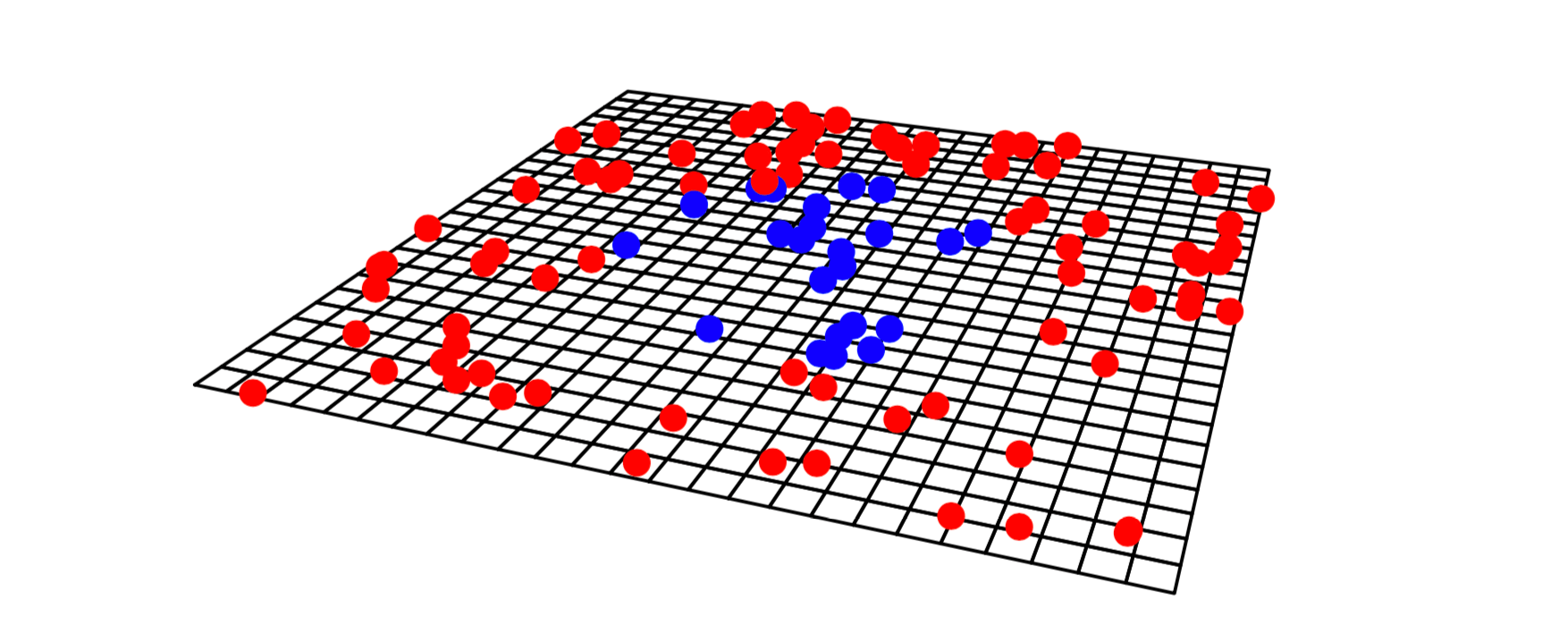It might be difficult, here, because which want to find a straight line in the two dimensional space $(x_1,x_2)$. But maybe, we can distort the space, possible by adding another dimension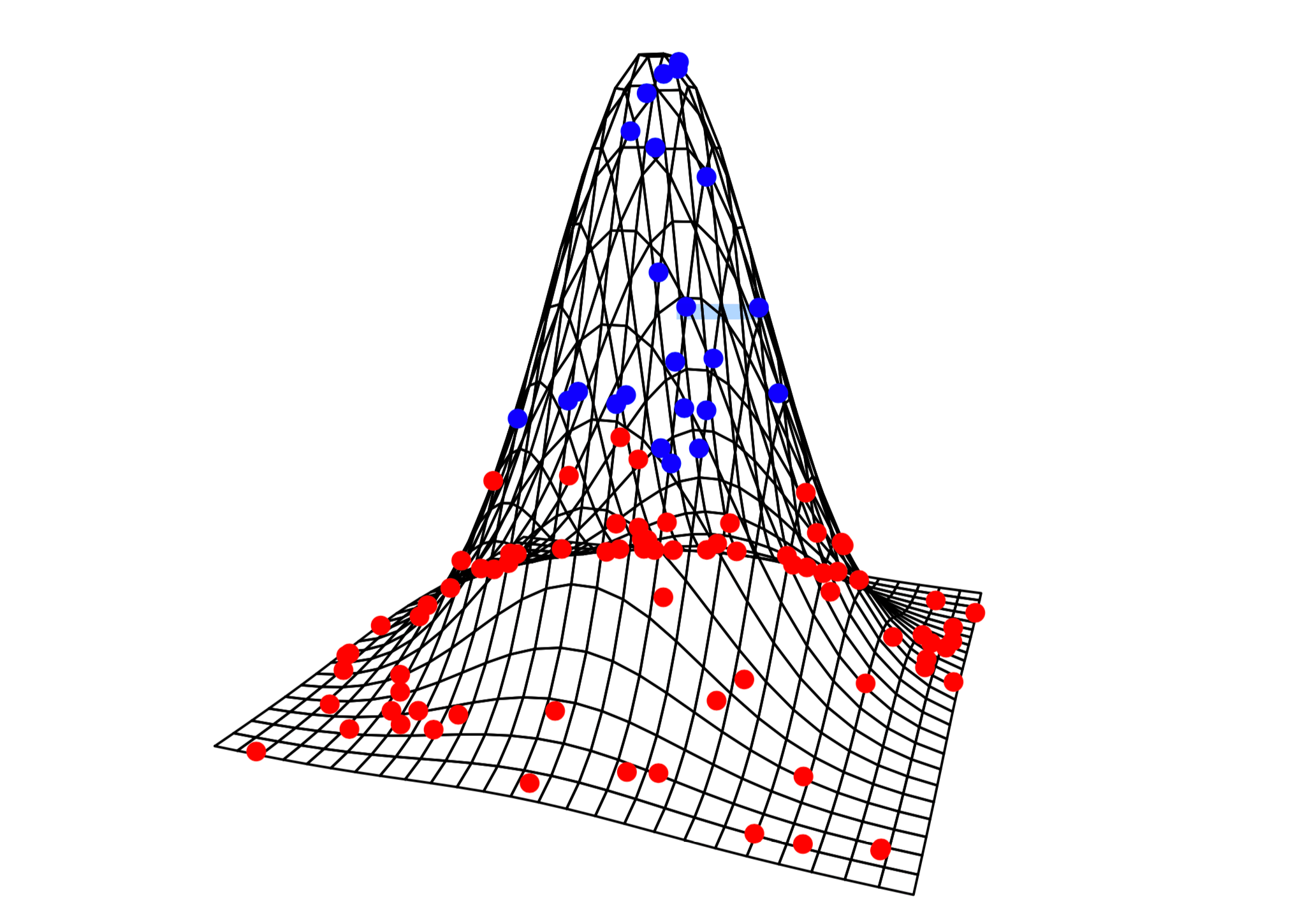That’s heuristically the idea. Because on the case above, in dimension 3, the set of points is now linearly separable. And the trick to do so is to use a kernel. The difficult task is to find the good one (if any).

A positive kernel on $\mathcal{X}$ is a function $K:\mathcal{X}\times\mathcal{X}\rightarrow\mathbb{R}$ symmetric, and such that for any $n$, $\forall\alpha_1,\cdots,\alpha_n$ and $\forall\mathbf{x}_1,\cdots,\mathbf{x}_n$,$$\sum_{i=1}^n\sum_{j=1}^n\alpha_i\alpha_j k(\mathbf{x}_i,\mathbf{x}_j)\geq 0.$$
For example, the linear kernel is $k(\mathbf{x}_i,\mathbf{x}_j)=\mathbf{x}_i^T\mathbf{x}_j$. That’s what we’ve been using here, so far. One can also define the product kernel $k(\mathbf{x}_i,\mathbf{x}_j)=\kappa(\mathbf{x}_i)\cdot\kappa(\mathbf{x}_j)$ where $\kappa$ is some function $\mathcal{X}\rightarrow\mathbb{R}$.

Finally, the Gaussian kernel is $k(\mathbf{x}_i,\mathbf{x}_j)=\exp[-\|\mathbf{x}_i-\mathbf{x}_j\|^2]$.

Since it is a function of $\|\mathbf{x}_i-\mathbf{x}_j\|$, it is also called a radial kernel.

linear.kernel = function(x1, x2) {
return (x1%*%x2)
}
svm.fit = function(X, y, FUN=linear.kernel, C=NULL) {
n.samples = nrow(X)
n.features = ncol(X)
K = matrix(rep(0, n.samples*n.samples), nrow=n.samples)
for (i in 1:n.samples){
for (j in 1:n.samples){
K[i,j] = FUN(X[i,], X[j,])
}
}
Dmat = outer(y,y) * K
Dmat = as.matrix(nearPD(Dmat)$mat) dvec = rep(1, n.samples) Amat = rbind(y, diag(n.samples), -1*diag(n.samples)) bvec = c(0, rep(0, n.samples), rep(-C, n.samples)) res = solve.QP(Dmat,dvec,t(Amat),bvec=bvec, meq=1) a = res$solution
bomega = apply(a*y*X,2,sum)
return(bomega)
}

To relate this duality optimization problem to OLS, recall that $y=\mathbf{x}^T\mathbf{\omega}+\varepsilon$, so that $\widehat{y}=\mathbf{x}^T\widehat{\mathbf{\omega}}$, where $\widehat{\mathbf{\omega}}=[\mathbf{X}^T\mathbf{X}]^{-1}\mathbf{X}^T\mathbf{y}$
But one can also write $$y=\mathbf{x}^T\widehat{\mathbf{\omega}}=\sum_{i=1}^n \widehat{\alpha}_i\cdot \mathbf{x}^T\mathbf{x}_i$$
where $\widehat{\mathbf{\alpha}}=\mathbf{X}[\mathbf{X}^T\mathbf{X}]^{-1}\widehat{\mathbf{\omega}}$, or conversely, $\widehat{\mathbf{\omega}}=\mathbf{X}^T\widehat{\mathbf{\alpha}}$.

## Application (on our small dataset)

One can actually use a dedicated R package to run a SVM. To get the linear kernel, use

library(kernlab)
df0 = df
df0$y = 2*(df$y=="1")-1
SVM1 = ksvm(y ~ x1 + x2, data = df0, C=.5, kernel = "vanilladot" , type="C-svc")

Since the dataset is not linearly separable, there will be some mistakes here

table(df0$y,predict(SVM1)) -1 1 -1 2 2 1 1 5 The problem with that function is that it cannot be used to get a prediction for other points than those in the sample (and I could neither extract $\omega$ nor $b$ from the 24 slots of that objet). But it’s possible by adding a small option in the function SVM2 = ksvm(y ~ x1 + x2, data = df0, C=.5, kernel = "vanilladot" , prob.model=TRUE, type="C-svc") With that function, we convert the distance as some sort of probability. Someday, I will try to replicate the probabilistic version of SVM, I promise, but today, the goal is just to understand what is done when running the SVM algorithm. To visualize the prediction, use pred_SVM2 = function(x,y){ return(predict(SVM2,newdata=data.frame(x1=x,x2=y), type="probabilities")[,2])} plot(df$x1,df$x2,pch=c(1,19)[1+(df$y=="1")],
cex=1.5,xlab="",
ylab="",xlim=c(0,1),ylim=c(0,1))
vu = seq(-.1,1.1,length=251)
vv = outer(vu,vu,function(x,y) pred_SVM2(x,y))
contour(vu,vu,vv,add=TRUE,lwd=2,nlevels = .5,col="red")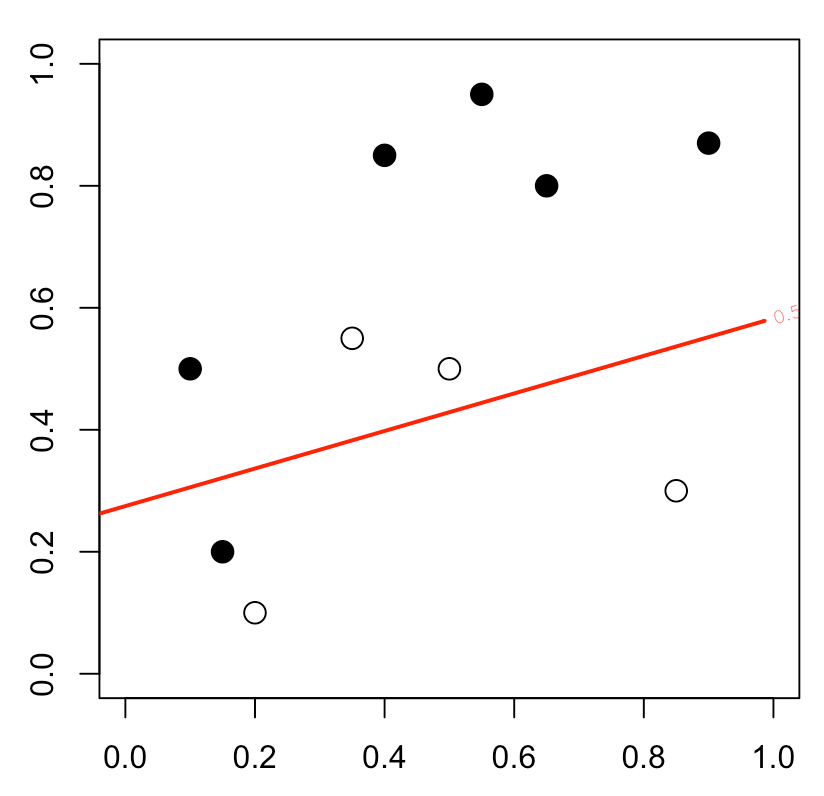Here the cost is $C$=.5, but of course, we can change it

SVM2 = ksvm(y ~ x1 + x2, data = df0, C=2, kernel = "vanilladot" , prob.model=TRUE, type="C-svc")
pred_SVM2 = function(x,y){
return(predict(SVM2,newdata=data.frame(x1=x,x2=y), type="probabilities")[,2])}
plot(df$x1,df$x2,pch=c(1,19)[1+(df$y=="1")], cex=1.5,xlab="", ylab="",xlim=c(0,1),ylim=c(0,1)) vu = seq(-.1,1.1,length=251) vv = outer(vu,vu,function(x,y) pred_SVM2(x,y)) contour(vu,vu,vv,add=TRUE,lwd=2,levels = .5,col="red")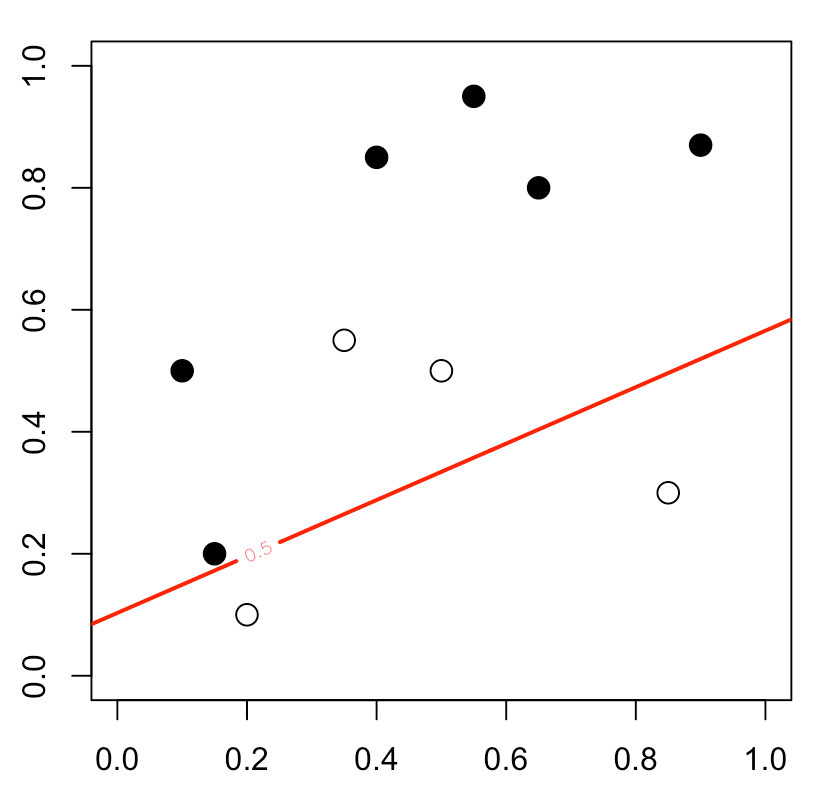As expected, we have a linear separator. But slightly different. Now, let us consider the “Radial Basis Gaussian kernel” SVM3 = ksvm(y ~ x1 + x2, data = df0, C=2, kernel = "rbfdot" , prob.model=TRUE, type="C-svc") Observe that here, we’ve been able to separare the white and the black points table(df0$y,predict(SVM3))

-1 1
-1  4 0
1   0 6
plot(df$x1,df$x2,pch=c(1,19)[1+(df\$y=="1")],
cex=1.5,xlab="",
ylab="",xlim=c(0,1),ylim=c(0,1))
vu = seq(-.1,1.1,length=251)
vv = outer(vu,vu,function(x,y) pred_SVM3(x,y))
contour(vu,vu,vv,add=TRUE,lwd=2,levels = .5,col="red")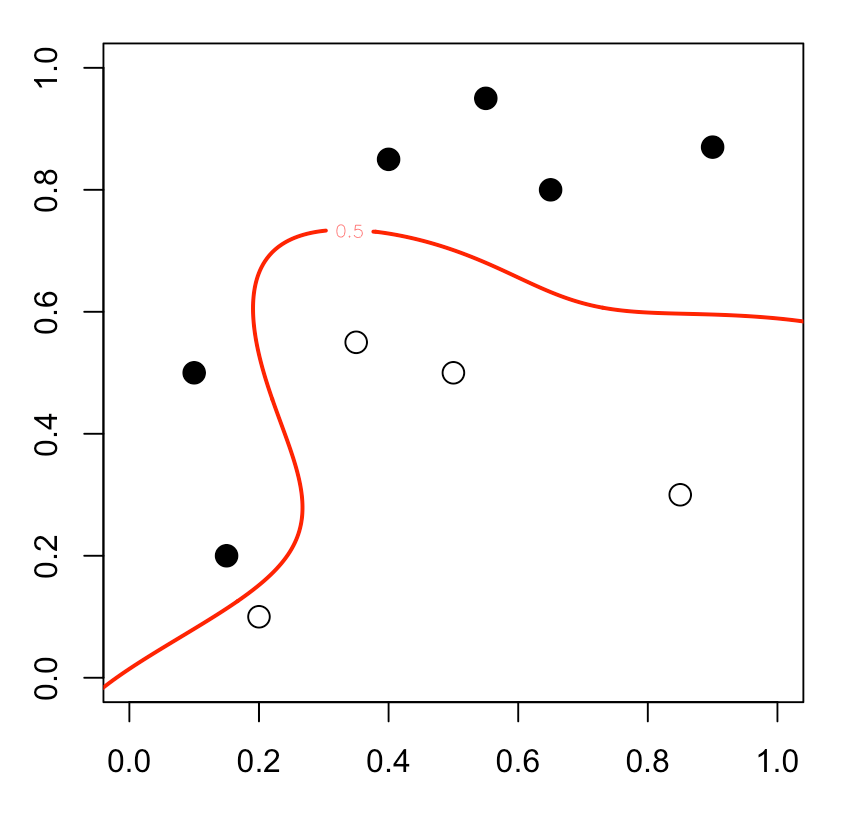Now, to be completely honest, if I understand the theory of the algorithm used to compute $\omega$ and $b$ with linear kernel (using quadratic programming), I do not feel confortable with this R function. Especially if you run it several times… you can get (with exactly the same set of parameters)or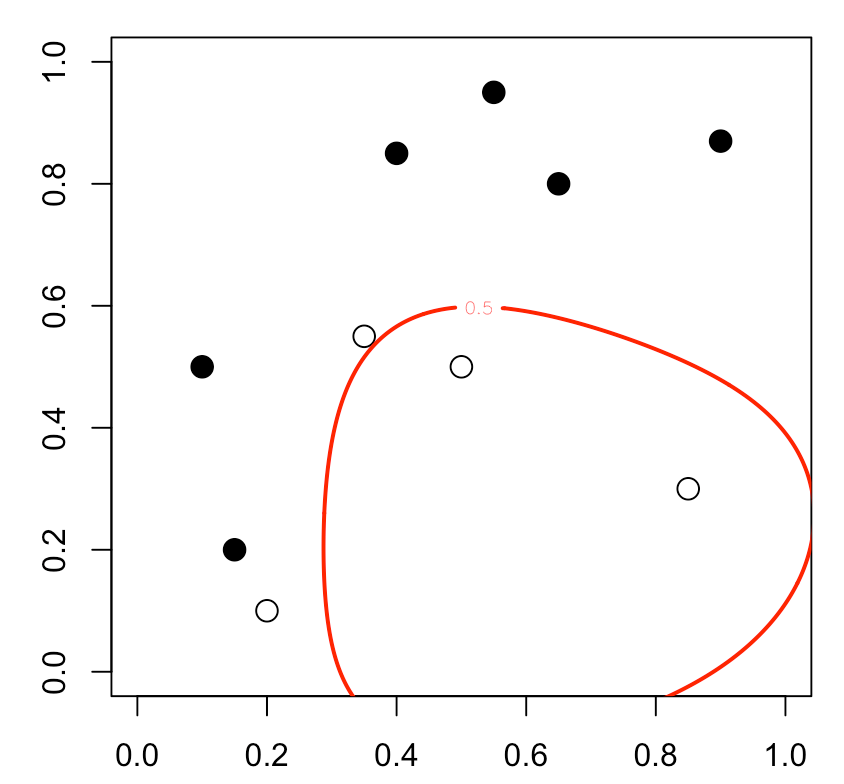(to be continued…)